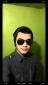# thermo

1 post / 0 new
zelRach02thermo

A spherical container of negligible thickness holding a hot fluid at 1400
and having
an outer diameter of 0.4 m is insulated with three layers of each 50 mm thick
insulation of k1 = 0.02: k2 = 0.06 and k3 = 0.16 W/mK. (Starting from inside). The
outside surface temperature is 300C. Determine (i) the heat loss, and (ii) Interface
temperatures of insulating layers.

need explaination plss..

• Mathematics inside the configured delimiters is rendered by MathJax. The default math delimiters are $$...$$ and $...$ for displayed mathematics, and $...$ and $...$ for in-line mathematics.